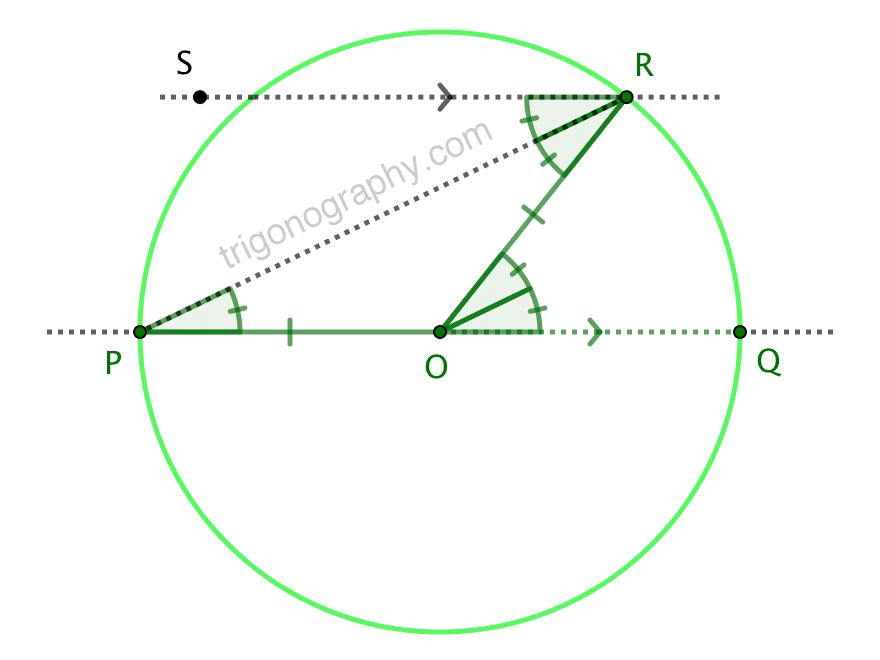## The Inscribed Angle Theorem without the Triangle Angle Sum Theorem

via hyperpallium in this question on the Mathematics Stack ExchangeOf course, $$\overline{OP}\cong\overline{OR}\;\Leftrightarrow\;\angle RPO = \angle PRO$$. And then

$$\angle RPQ = \angle PRS \quad\Leftrightarrow\quad \overleftrightarrow{PQ}\parallel\overleftrightarrow{RS}\quad\Leftrightarrow\quad\angle ROQ = \angle ORS = 2\angle RPQ$$

This base case of the Inscribed Angle Theorem is typically proven by way of the
Triangle Angle Sum Theorem (perhaps in the form of the “strong” Exterior Angle Theorem).
After all, what does anyone remember from (Euclidean) Geometry class, except that
“The angles of a triangle add-up to $$180^\circ$$” (and maybe the Pythagorean Theorem)?
But the TAST, in turn, is typically proven by way of the Alternate Interior Angles
Theorem for parallel lines. (The results are, in fact, logically equivalent.)

hyperpallium has demonstrated that such a digression is actually unnecessary.
The inscribed angle property follows nicely from the parallelism property. Nifty!